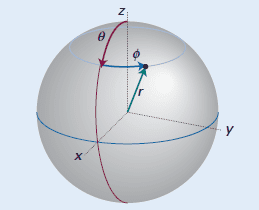# Deriving the Laplacian in spherical coordinates

Mayhem
Homework Statement:
Derive the Laplacian in spherical coordinates using the Laplacian in rectangular coordinates
Relevant Equations:
##\nabla = \left ( \frac{\partial }{\partial x}, \frac{\partial }{\partial y}, \frac{\partial }{\partial z}\right )##

##x=r \sin(\theta) \cos(\phi), y=r \sin(\theta) \sin(\phi), z = r \cos(\theta)##
As a part of my self study, I am trying to derive the Laplacian in spherical coordinates to gain a deeper understanding of the mathematics of quantum mechanics.
For reference, this the sphere I am using, where ##r## is constant and ##\theta = \theta (x,y, z), \phi = \phi(x,y)##.Given the definitions in "Relevant Equations", we can define the partial derivatives of ##x, y## and ##z##\begin{align*} \frac{\partial }{\partial x} &= r\left(\cos(\theta)\cos(\phi)\frac{\partial }{\partial \theta} - \sin(\theta)\sin(\phi)\frac{\partial }{\partial \phi}\right ) \\ \frac{\partial }{\partial y} &= r\left(\cos(\theta)\sin(\phi)\frac{\partial }{\partial \theta} - \sin(\theta)\cos(\phi)\frac{\partial }{\partial \phi}\right ) \\ \frac{\partial }{\partial z} &= -r \sin(\theta) \frac{\partial }{\partial \theta} \end{align*}
Thus the gradient in spherical coordinates becomes $$\nabla = \begin{pmatrix} r\left(\cos(\theta)\cos(\phi)\frac{\partial }{\partial \theta} - \sin(\theta)\sin(\phi)\frac{\partial }{\partial \phi}\right )\\ r\left(\cos(\theta)\sin(\phi)\frac{\partial }{\partial \theta} - \sin(\theta)\cos(\phi)\frac{\partial }{\partial \phi}\right ) \\ -r \sin(\theta) \frac{\partial }{\partial \theta}\end{pmatrix}$$
The Laplacian is given as ##\Delta = \nabla \cdot \nabla = \nabla^2##.

My question is: once I take the dot product of the given gradient vector, will I be able to simplify into the Laplacian for spherical coordinates? I am asking, because it takes a lot of brute calculations to find out, and therefore I want to know if my initial math is correct.

•Delta2

Homework Helper
Gold Member
2022 Award
You've expressed the partial derivatives in terms of ##\theta## and ##\phi##, but you've omitted the derivatives in terms of ##r##. Also, the vector is still in terms of the Cartesian basis vectors, rather than ##\hat r## etc.

The Laplacian has second derivatives with respect to ##x, y, z##, so you should expect second derivatives with respect to ##r, \theta, \phi## to appear.

So, you have some mistakes and misconceptions, I'm sorry to say.

•docnet
Mayhem
You've expressed the partial derivatives in terms of ##\theta## and ##\phi##, but you've omitted the derivatives in terms of ##r##. Also, the vector is still in terms of the Cartesian basis vectors, rather than ##\hat r## etc.

The Laplacian has second derivatives with respect to ##x, y, z##, so you should expect second derivatives with respect to ##r, \theta, \phi## to appear.

So, you have some mistakes and misconceptions, I'm sorry to say.
Where exactly do I start then?

Homework Helper
Gold Member
2022 Award
Where exactly do I start then?
I just noticed that your derivatives are not right:

Given the definitions in "Relevant Equations", we can define the partial derivatives of ##x, y## and ##z##\begin{align*} \frac{\partial }{\partial x} &= r\left(\cos(\theta)\cos(\phi)\frac{\partial }{\partial \theta} - \sin(\theta)\sin(\phi)\frac{\partial }{\partial \phi}\right ) \\ \frac{\partial }{\partial y} &= r\left(\cos(\theta)\sin(\phi)\frac{\partial }{\partial \theta} - \sin(\theta)\cos(\phi)\frac{\partial }{\partial \phi}\right ) \\ \frac{\partial }{\partial z} &= -r \sin(\theta) \frac{\partial }{\partial \theta} \end{align*}
It's generally better to included test function to avoid mistakes:
$$\frac{\partial f }{\partial x} = \frac{\partial f}{\partial r}\frac{\partial r}{\partial x} + \frac{\partial f}{\partial \theta}\frac{\partial \theta}{\partial x} + \frac{\partial f}{\partial \phi}\frac{\partial \phi}{\partial x}$$

Mayhem
I just noticed that your derivatives are not right:

It's generally better to included test function to avoid mistakes:
$$\frac{\partial f }{\partial x} = \frac{\partial f}{\partial r}\frac{\partial r}{\partial x} + \frac{\partial f}{\partial \theta}\frac{\partial \theta}{\partial x} + \frac{\partial f}{\partial \phi}\frac{\partial \phi}{\partial x}$$
I am a little confused it seems. I cannot take ##r## to be constant?

Homework Helper
Gold Member
2022 Award
I am a little confused it seems. I cannot take ##r## to be constant?
Definitely not. ##r## is one of the three coordinates.

Mayhem
Definitely not. ##r## is one of the three coordinates.
I see. I found this and it seems what I am trying to accomplish isn't exactly a simple task (probably why my textbook omitted the derivation), but I will try to work through it.

Homework Helper
Gold Member
2022 Award
I see. I found this and it seems what I am trying to accomplish isn't exactly a simple task (probably why my textbook omitted the derivation), but I will try to work through it.
That's even more work than I recall. There are quicker ways to do it using results for general coordinate systems.

Mayhem
That's even more work than I recall. There are quicker ways to do it using results for general coordinate systems.
Hm.

I think I might have to backtrack a little and consider the same problem but in two dimensions, and then once I understand that, I can move on to the 3D case.